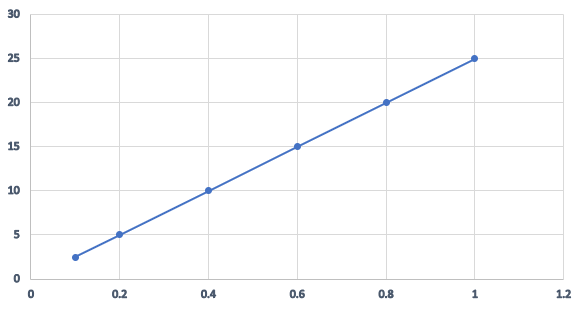# (a) What is the ratio of potential difference and current known as? (b) The values of potential difference $V$ applied across a resistor and the correponding values of current $I$ flowing in the resistor are given below: Potential difference, $V$ (in volts)  :  $\begin{array}{llllll}2.5 & 5.0 & 10.0 & 15.0 & 20.0 & 25.0\end{array}$Current, $I$ (in amperes)        :  $\begin{array}{llllll}0.1 & 0.2 & 0.40 & 0.60 & 0.80 & 1.0\end{array}$Plot a graph between $V$ and $I$, and calculate the resistance of the resistor.(c) Name the law which is illustrated by the above $V-I$ graph.(d) Write down the formula which states the relation between potential difference, current and resistance.(e) The potential difference between the terminals of an electric iron is $240 \mathrm{~V}$ and the current is $5.0 \mathrm{~A}$. What is the resistance of the electric iron?

(a) The ratio of potential difference and current is known as resistance.

(b)
Values of voltage should be plotted on the Y-axis and values of current should be plotted on the X axis.According to ohm's law:
$\mathrm{R}=\frac{\mathrm{V}}{\mathrm{I}}$
From the graph, resistance, $\mathrm{R}=\frac{V}{I}$
$R =\frac{5}{0.2}$
$=25 \Omega$

(c) Ohm's law is represented through $V$ and $I$ graph for a conductor. Since the graph is a straight line, we can conclude that $V \propto I$.

Ohm's law states that at a constant temperature, the current passing through a conductor is directly proportional to the potential difference applied across it.

(d) According to ohm's law:

$\mathrm{I}=\frac{\mathrm{V}}{\mathrm{R}}$. This gives the relation between potential difference V, current I and resistance R.

(e)

According to ohm's law:

$\mathrm{R}=\frac{\mathrm{V}}{\mathrm{I}}$

$\mathrm{R}=\frac{\mathrm{240}}{\mathrm{5}}$

So we get, Resistance R = 48 ohm

Updated on: 10-Oct-2022

615 Views